## The Laws Of Refraction

The Laws of Refraction

• Incident ray, reflected ray, refracted ray and the normal of the system lie in the same plane.
• Incident ray, coming from one medium to the boundary of another medium, is refracted with a rule derived from a physicist Willebrord Snellius. He found that there is a constant relation between the angle of incident ray and angle of refracted ray. This constant is the refractive index of second medium relative to the first medium. He gives the final form of this equation like;
•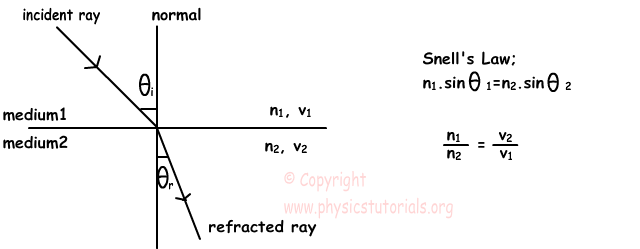Where n1 is the refractive index of first medium and n2 is the refractive index of second medium, v1 is the speed of light in firs medium and v2 is the speed of light in second medium.

Example: Find the velocity of the ray in a medium having refractive index 2.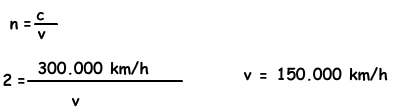Example: A ray coming from medium X is refracted as shown in the figure below while passing to the medium Y. Find ratio of the refractive indexes of the mediums. (sin37º=0, 6 and sin53º=0, 8)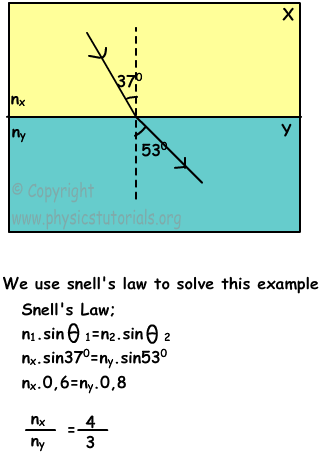• When we give the refractive index of medium you should understand that it is the relative refractive index of that medium.

• In general, if the density of the medium increases than refractive index of that medium also increases. However, of course there are some exceptions like water.

• Velocity of the light in a medium is inversely proportional to the refractive index of that medium. If the refractive index increases then velocity of the light decreases.

• If the light comes perpendicular to the boundary of two different mediums, it does not change its direction. Because it is on the normal line of the system. But, the velocity of the light changes since the density of the medium changes.

• Refractive index of the medium is also depends on the color of the coming light. For example, refractive index of the medium for violet colors is larger than the refractive index of the medium for other colors.

• The angle of refraction of the light coming from the medium having smaller refractive index is smaller than the angle of incident ray. Look at the given diagram that shows this relation.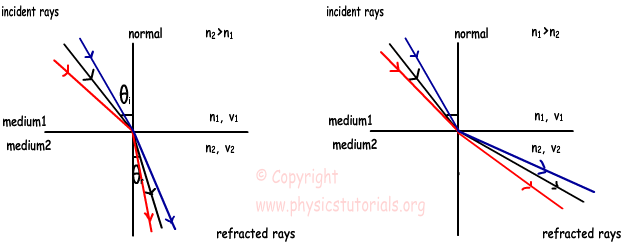Example: Find the path of the ray after refracting.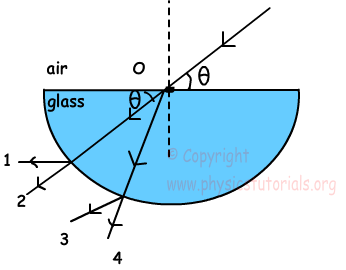Ray coming from air to glass is refracted from the surface, thus 1 and 2 choices are eliminated. When the light comes to the boundary of the glass it does not refract since it comes from the normal of the system and goes in a straight line. Thus, it follows the path shown in 4.

Optics Exams and Solutions

Author: